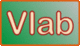online_muskingum_cunge_convergence_ratios:    Convergence ratios of the Muskingum-Cunge method

INPUT DATA:            [How to use]

Weighting factor X :

[Recommended range:  0.0 ≤ X ≤ 0.5]

Spatial resolution Lx :

[Recommended range:  20 ≤ Lx ≤ 100]

Courant number C :

[Recommended range:  0.1 ≤ C ≤ 4.0]Formulas: σΔx = 2π/(L/Δx) p = cos(σΔx) q = sin(σΔx) R = X + 0.5 C S = R - C M = (1 - p)(1 - 2S)C N = qC P = 1 - 2[(1 - p)(1 - S)S] R1 = [(P - M)2 + N 2]1/2 / P R2 = [1/(C σΔx)] tan-1[N/(P - M)] Reference OUTPUT: Amplitude convergence ratio R1 :  0 Phase convergence ratio R2 :  0

Go to ⇒   Online Muskingum-Cunge convergence ratios - practical

Your request was processed at  09:53:41 pm on June 30th, 2022   [ 220630  21:53:41 ].

Thank you for running online_muskingum_cunge_convergence_ratios